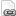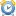# CONCEPT - MATHEMATICAL MODELS

Home > The Ocean is Largely Unexplored > Mathematical Models

## Aquatic Model Mania - COSEE Coastal TrendsSummary
Using a simulated model, students observe and manipulate the interactions of the components of an aquatic food web based upon the availability of nutrients.

Concept: Mathematical models
Mathematical models are an essential tool for understanding ocean sciences.

Education Standards45 mins

## Case Study: Upwelling - Monterey Bay Aquarium Research InstituteSummary
Students correlate sardine populations changes with upwelling events using mathematical model output and wind and water circulation data from the Monterey Bay.

Concept: Mathematical models
Mathematical models are an essential tool for understanding ocean sciences.

Education Standards45 mins